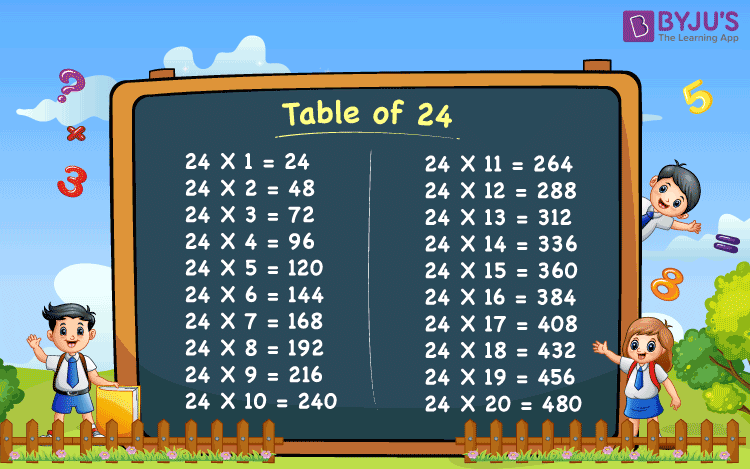# Table of 24

In this, we will read and memorize the table of 24. You can also download the pdf of the 24 times table image from here for further reference. Table of 24 helps us to solve mathematical problems easily and quickly. The tables are an essential part of students’ life. Especially, the primary class students whose most of the syllabus consists of arithmetic calculations, tables play an important role. Not only for academic exams but also for competitive exams, these tables are beneficial.

## Table of 24 Chart## 24 Times Table

 24 X 1 = 24 24 X 2 = 48 24 X 3 = 72 24 X 4 = 96 24 X 5 = 120 24 X 6 = 144 24 X 7 = 168 24 X 8 = 192 24 X 9 = 216 24 X 10 = 240 24 X 11 = 264 24 X 12 = 288 24 X 13 = 312 24 X 14 = 336 24 X 15 = 360 24 X 16 = 384 24 X 17 = 408 24 X 18 = 432 24 X 19 = 456 24 X 20 = 480

Forward counting : 24, 48, 72, 96, 120, 144, 168, 192, 216, 240, 264, 288, 312, 336, 360, 384, 408, 432, 456, 480.

Backward counting : 480, 456, 432, 408, 384, 360, 336, 312, 288, 264, 240, 216, 192, 168, 144, 120, 96, 72, 48, 24.

## Related Articles

Let’s look at the tables here.

## Solved Examples on Table of 24

(i) When 2 packs each having 24 pastel colours.

By repeated addition we can show 24 + 24 = 48

Then, 2 times of twenty-four is,

2 × 24 = 48

Therefore, there are 48 pastel colours.

(ii) When 3 bunches of 24 bananas each.

By repeated addition we can show 24 + 24 + 24 =72. Then, twenty-four times  3 is

3 × 24 = 72

Therefore, there are 72 bananas.

(iii) When 4 bunches of 24 bananas each.

By repeatedly adding we can show 24 + 24 + 24 +24 = 96

Then, twenty-four times 4 is

4 × 24 = 96

Therefore, there are 96 bananas.

(iv) When 5 bunches of 24 bananas each.

By repeated addition we can show 24 + 24 + 24 + 24 +24 = 120

Then, twenty-four times 5 is

5 × 24 = 120

Therefore, there are 120 bananas.

(v) When 6 bunches of 24 bananas each.

By repeated addition we can show 24 + 24 + 24 + 24 + 24 + 24 = 144

Then, twenty-four times 6 is

6 × 24 = 144

Therefore, there are 144 bananas.

(vi)When 7 bunches of 24 bananas each.

By repeated addition we can show 24 + 24 + 24 + 24 + 24 + 24 +24 = 168

Then, twenty-four times 7 is

7 × 24 = 168

Therefore, there are 168 bananas.

(vii) When 8 bunches of 24 bananas each.

By repeatedly adding we can show 24 + 24 + 24 + 24 + 24 + 24 + 24 + 24 = 192

Then, twenty-four times 8 is

8 × 24 = 192

Therefore, there are 192 bananas.

(viii) When 9 bunches of 24 bananas each.

By repeated addition we can show 24 + 24 + 24 + 24 + 24 + 24 + 24 + 24 +24 = 216

Then, twenty-four times 9 is

9 × 24 = 216

Therefore, there are 216 bananas.

(ix) When 10 bunches of 24 bananas each.

By repeated addition we can show 24 + 24 + 24 + 24 + 24 + 24 + 24 + 24 +24 +24 = 240

Then, twenty-four times 10 is

10 × 24 = 240

Therefore, there are 240 bananas.

Multiplication Tables are used in everyday life.

Visit BYJU’S to check out the other multiplication tables.

Test your knowledge on Table Of 24